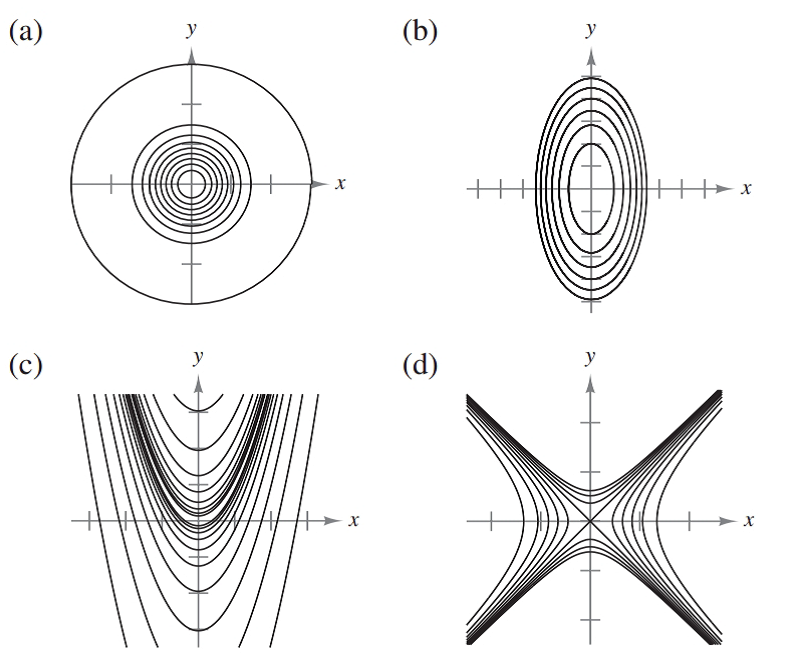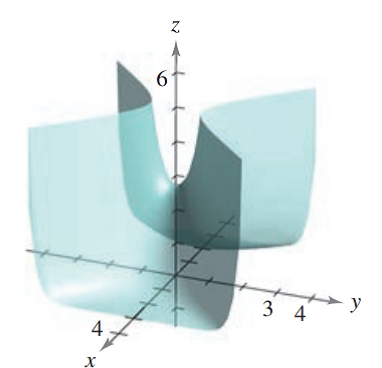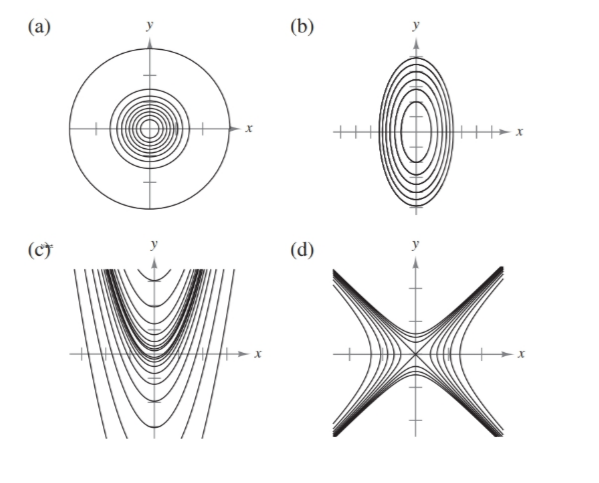Chapter 7.3, Problem 32E### Calculus: An Applied Approach (Min...

10th Edition
Ron Larson
ISBN: 9781305860919

#### Solutions

Chapter
Section### Calculus: An Applied Approach (Min...

10th Edition
Ron Larson
ISBN: 9781305860919
Textbook Problem
1 views

# Matching In Exercises 31-34, match the graph of the surface with one of the contour maps. [The contour maps are labeled (a)-(d).]f ( x , y ) = e 1 − x 2 + y 2To determine

The correct match for the function f(x,y)=e1x2+y2 from the following options:Explanation

Given Information:

The option of provide function f(x,y)=e1x2+y2 are:

The graph of equation f(x,y)=e1x2+y2 given below,

Consider the provide function,

f(x,y)=e1x2+y2

Substitute f(x,y)=C1 in the equation f(x,y)=e1x2+y2 to obtain contour map,

C1=e1x2+y2

Take log both sides,

lnC1=lne1x2+y21x2+y2=C11

This equation represent the equation of hyperbola.

Substitute f(x,y)=C2 in the equation f(x,y)=e1x2+y2 to obtain contour map,

C2=e1x2+y2

Take log both sides,

lnC2=lne1x2+y21x2+y2=C21

This equation represent the equation of hyperbola

### Still sussing out bartleby?

Check out a sample textbook solution.

See a sample solution

#### The Solution to Your Study Problems

Bartleby provides explanations to thousands of textbook problems written by our experts, many with advanced degrees!

Get Started